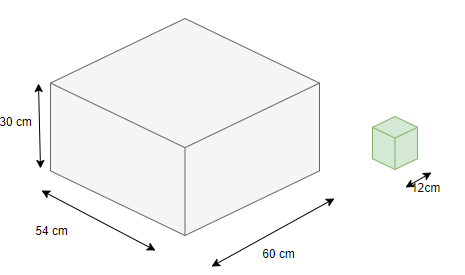# Extra questions for Mensurations

In this page we have Extra questions for Mensurations Class 8 Maths . Hope you like them and do not forget to like , social share and comment at the end of the page.
Question 1
Given a cuboid tank, in which situation will you find surface area and in which situation volume.
(a) To find how much it can hold.
(b) Number of paint bottle required to paint it.
(c) To find the number of smaller tanks that can be filled with water from it.

Question 2
Compare the volumes
(a) Cube (side =12 cm)
Cuboid (L=11 cm, B=12 cm, H=13 cm)
(b) Cylinder ( r=10 cm , H=14 cm)
Cuboid (L=10 cm, B=11 cm, H=14 cm)

Question 3
Find following
(a) the height of a cuboid whose base area is 180 cm2and volume is 900 cm3?
(b) The side of cube whose volume is 64 m3
(c) Volume of the cylinder whose base area is 20 cm2 and height is 10 cm

Question 4
A cuboid is of dimensions 60 cm × 54 cm × 30 cm. How many small cubes with side 12 cm can be placed in the given cuboid?Question 5
Find the height of the cylinder whose volume is 2.54 m³ and diameter of the base is 140 cm?

Question 6
A water tank is in the form of cuboid whose length is 1.5 m , height is 2 m and Breath is 7 m. Find the quantity of water in litres that can be stored in the tank?

Question 7
If each edge of a cube is quadrupled,
(i) how many times will its surface area increase?
(ii) how many times will its volume increase?

Question 8
Water is pouring into a cuboidal reservoir at the rate of 60 liters per minute. If the volume of reservoir is 108 m³, find the number of hours it will take to fill the reservoir.

Question 9
If Length, Breath, Height of a cuboid is tripled,
(i) how many times will its surface area increase?
(ii) how many times will its volume increase?

Question 10
If radius of cylinder is tripled and height remains same
(i) how many times will its surface area increase?
(ii) how many times will its volume increase?

## Summary

This Extra questions for Mensurations Class 8 Maths is prepared keeping in mind the latest syllabus of CBSE . This has been designed in a way to improve the academic performance of the students. If you find mistakes , please do provide the feedback on the mail.